# Computer Science notes ⇒ Principles of Programming

This module relies on the textbook "Structure & Interpretation of Computer Programs (2nd Edition)" by Abelson, Sussman and Sussman.

This module doesn't teach you how to program in a particular language, but how to program in general principles that can be applied to any language. A good analogy is that between linguistics and English. Linguistics teaches the principle of language, whereas English just teaches one particular language. However, as linguistics is taught in English, we also need to know a language to demonstrate programming skills in. Such a language is Scheme.

## Introduction to SchemeIn Scheme, numbers are expressions known as primitive expressions. Other expressions are compound (or combination) expressions.

Compound expressions always have the form: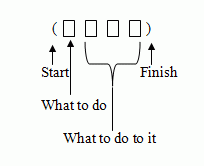Scheme uses prefix notation, for example: (+ 7 6) gives 13. Expressions can be also be nested. 2 + 3 * 4 is equivalent to (+ 2 (* 3 4)) in Scheme.

The value of a compound expression is the result of applying the procedure specified by the operator to the operands.

To avoid typing out long expressions multiple times when applying different procedures to it, you can use definitions, for example: (define name value). This

• Creates a new variable - allocates a memory location where values are stored.
• Gives the location a name and links the name to that variable.
• Puts the value in that location.

Variables are also primitive expressions, but they can be procedures.

Scheme stores the variables in a table of names and values called the environment.

SICP 1.1.3, 2.3.1

Evaluation is recursive. To evaluate a compound expressions:

1. Evaluate each sub-expression
2. Apply the values of the first sub-expression to the latter.

The first value of an expressions must be a procedure.

### Procedures and Abstraction

SICP p62

Procedures are defined using lambda. The value of (lambda) is the procedure, which when applied to a list of operand values evaluates body with substitutions for the corresponding parameters.

(lambda (param1 param2 ... paramn) (body) )

The params are zero or more parameters. body is an expression which refers to the parameters.

An alternative is: (define (name param1 param2 ... paramn) (body) ).

SICP 1.1.4

There are many different ways of defining equivalent procedures, so what is the best way to do so? Not using abstraction.

Abstraction is the generalisation of a method, e.g., defining double and then using that rather than (* 2 6). Abstraction is our fundamental design methodology.

### What Happens During Evaluation?

Take for example this code:

(define (double x) (* 2 x))
(define (triple x) (+ x (double x)))

SICP p13/14

Scheme uses the substitution model for evaluation, so evaluating some simple code goes like this:

(triple 5)

(+ 5 (double 5))
(+ 5 (*2 5))
(+ 5 10)

15

### Choice

#### either/or

SICP 1.1.6

You can do either/or choices like so: (if expression then else).

expression is evaluated to true or false.
then is the value of the if expression if the expression is true
else is the value of the if expression if the expression is false.

#f is false, and #t is true, however anything that isn't #f is considered to be true.

To add more power to choice, you can use logical composition operations.

(and expr1 expr2 ... exprn)

This is evaluated left to right, until one is false or all are true. This is different to the standard Scheme operation.

(or expr1 expr2 ... exprn)

This also evaluates left to right, until one is true or all are false.

(not expr)

This returns the opposite value to expr.

#### One of several

SICP p17

(cond (pred1 expr1) (pred2 expr2) ... (predn exprn) )

The value of (cond) is the value of the first expression whose predicate evaluates to true. If the predicate is #t, this is always true and is a useful "catch-all" situation.

### Evaluation

SICP ex1.5

#### Applicative vs Normal Order

Normal order substitutes expressions for parameters until left with primitive procedures only. Applicative does not evaluate the operands until the values were needed. Instead, it substitutes operand expressions for parameters until it obtained an expression involving only primitive operators and then evaluates it.

### Recursive Definition

All follow the same general pattern. It consists of both a base case (or cases), where the value does not refer to the procedure and a recursive case, where the value uses the procedure. Which case it is is determined by the values of the parameters.

(sequence) allows you to chain together multiple procedures, and the value is the value of the last expression.

(print) is a special form with a side-effect. It prints a value to the terminal but does not effect the environment in any way.

### Compound Data

 Procedures Data + - quote evaluate to » primitive (built in) « evaluates to numbers, symbols, booleans (lambda) compound (built from primitive and other compound) ?

As the ? suggests, you can have compound data, such as lists.

#### Lists

SICP 2.2.1, p101 (footnote 10)

There are 2 forms of lists, empty and non-empty. Non-empty lists are values followed by another list. An empty list is the value nil.

##### Constructors

(cons) allows you to create lists, like so: (cons expr list). This creates a list consisting of the value of expr followed by list. Lists can also have other lists as values (sublists)

##### Selectors

(car list) returns the first element (head) of a list.

(cdr list) returns the list without the first element (tail).

There are also shortcuts, such as (cadr list), which is equivalent to (car (cdr list)). There are also (caddr list), (cadar list), etc.

There are also some tests (predicates) which can be applied to lists. (null? expr) is true is the expr is nil. (list? expr) is true if expr is a list.

##### Useful list procedures

SICP p100

(list expr expr)

Creates a list containing the values of the expressions

SICP p103

(append list1 list2)

Creates a list containing all the elements of list1, followed by all of the elements of list2

SICP p102

(length list)

This is the number of elements in the list.

### Local Procedure Definitions

The body of a procedure definition can take the form:

(define ...) (define ...) (expr)

The definitions bind names to values. Then expr is evaluated in a local environment in which these bindings are valid.

SICP 1.1.8

expr is said to be in scope of these bindings.

(let ((name value) (name value) ...) expr)

The value of the (let) expression is the value of expr in the environment in which each name corresponds to a value. This avoids evaluating the same code repeatedly.

SICP p63-66

N.B. expr is any expression, including another (let). (let)s can be nested.

## Computational Processes

A process is represented by a sequence of operations that are generated by a procedure when it is evaluated.Memory is stored in the stack. The stack space required for our our factorial program is proportional to n, where n is the number to be computed. We say this is of order n, or O(n). The time complexity is also O(n)

SICP 1.2.3

O(something) resource means roughly: "resource is used up no faster than something as the problem size increases"

### Factorial Iteratively

To do factorial iteratively, we need to keep a note of the answer so far and a counter which goes up from 1. This gets passed as parameters in our recursion. When counter > n, the final answer is the same as the answer so far. This has a space complexity is O(1). Any procedure that has a space complexity of O(1) is called iterative.

### Tail Recursion

SICP p35

A procedure that is tail-recursive if the recursive call is not a sub-expression of some other expression in the procedure body. Informally, something is tail recursive is a recursive call is the last thing done by the procedure. Tail recursive procedures generate iterative processes (O(1) space complexity)

### Complexity

SICP 1.2.2

 Time Space Recursive O(kn) O(kn) "exponential" Iterative O(n) O(1)

## Abstraction

See: Dictionary

1. Separated from matter, practice or particular examples.
2. Ideal or theoretical way of regarding things

#### Abstract (verb)

remove, summarise

#### Abstraction (noun)

Process of stripping an idea from its concrete accompaniment

## First class elements

SICP 1.3.1-1.3.3, p58

An element is a first class element in a language if it can be:

• named by variables
• passed as arguments to procedures
• returned as the results of procedures
• included in data structures

## Abstract Data Types

ADTs are defined by a set of interface procedures.

• Constructors - make instances of data
• Selectors - returns value of the components of the data
• Type predicates - test whether something is an instance of the data type

For example, lists are a built in ADT in Scheme. You have constructors (cons, nil), selectors (car, cdr) and type predicates (list?, null?) .

Using this type of abstraction allows you to split the load of creating a system.

## Types

A type is a set of values (and operations, procedures, etc - e.g., eq? a b). x :: t means x is a type of t. e.g., in Scheme:

 numbers 42 10.5 -7 1e-4 symbols 'h 'thing booleans #t #f pairs (2 . 3) (cons 2 3) procedures # strings "hello world" nil '() unspecified # characters

The union of all of these types could be called Scheme values.

### Type Constructors

Product: A x B is the type of a pair of values, the first of type A and second of type B.
Union: A + B is the type of a value of type A or of type B.
Function: A -> B is the type of a procedure which takes a value of type A and returns a value of type B.

#### (+ a b)

(Number x Number) --> Number

#### (sigma f a b)

sigma :: (number --> number) x number x number --> number

#### (combine-with operator identity f a b)

combine-with :: ((number x number) --> number) x number x (number --> number) x number x number --> number

#### ax2 + bx + c

quadratic :: number x number x number --> (number --> number)

### Type Checking

#### Static

• All types checked before program is run (compile time)
• Most languages do this
• This is safer and has no runtime overhead

#### Dynamic

• Types checked when an expression is evaluated (at run-time)
• Scheme does this
• This is more flexible

Functional languages (Haskell, ML, etc) exist that combine both. They have very powerful static type checking, the best of both worlds.

## Representing Objects

### Assignment

SICP 3.1

(set! name expression)

Changes the value bound to a name to the value of an expression. The name must already be defined and exist in the environment which set! is evaluated.

### The Substitution Model

SICP 1.1.5

To evaluate a compound procedure, evaluate the body of the procedure with each formal parameter replaced by the value of the corresponding argument.

SICP 3.2

There are problems with global variables to store state. There may be multiple instances of items and global variables decrease modularity. To work round this, you can encapsulate state within an object. An object must have internal state and be active. You tell the object what to do with the state rather than do it directly.

In Scheme, objects are represented by procedures with local state variables.

• functional
• imperative
• logic
• object-orientated (sometimes called message-passing)

### The Environment Model of Evaluation

The substitute model doesn't work when assignment is involved.

An environment is made of frames, where frames are tables of bindings (names and values) and a pointer to the enclosing environment. In a frame, a variable can be bound, unbound, the first binding or a "shadow" binding (overriding any earlier bindings).

A procedure is the code and pointer to an environment. This is the environment in which the lambda expression defining the pointer was evaluated.

e.g., "blood-donor" is an object which has state (quantity of blood) and responds to "drain" requests. A constructor of blood donors takes a quantity of blood and returns a blood donor object. This object is itself a procedure which responds to requests (drain, etc) and then returns the procedure to do that event, which itself may take multiple arguments.

An object needs to be defined with initial states, the object procedure and request handling procedures. The object constructor should return the object.

### Mutators

 Primitive Data Compound Data Creation 1, 'x, #f Constructors cons Selectors car, cdr Assignment set! Mutators set-car!, set-cdr!

## Pointers

### Box and Pointer Diagrams

SICP 2.2, 2.3

(cons x y) creates a new pair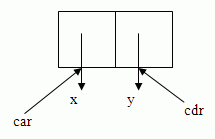The contents of the pair are pointers.

A list terminator can be represented as follows: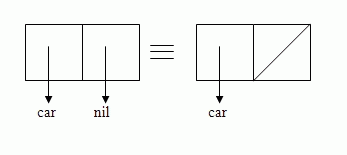e.g., '(1 2 3) is the short form of (cons 1 (cons 2 (cons 3 (nil))))### Equality

SICP p257-258

=, two numerical values are the same
eq?, two pointers are the same
equal?, two structures are the same (here, it is the representation of the structures that are compared)

Say we create 2 structures, (cons x 'a 'b) and (cons z1 x x)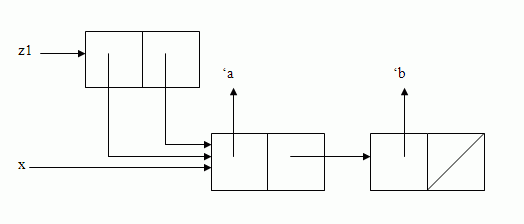We can now do (set-car! (cdr z1) 'boom) which changes 'a in the structure to 'boom. This now makes z1 (('boom 'b) 'boom 'b) and x is now ('boom 'b). However, if we (cons z2 (list 'a 'b) (list 'a 'b)), this makes z1 equal? to z2, but now (set-car! (cdr z2) 'boom) gives us (('a 'b) 'boom 'b)

### Association List

An association list is a list of records, each of which consists of a key and a value. Each record is a pair formed by (cons key value).

e.g., ((answer . 23) ('y . #t) ('z . "Hello")) associates answer with 23, y with #t and z with "Hello".

The procedure (retrieve key associations) returns the first pair in associations which has key in its car or returns nil if there is no such record.

### Tables

SICP 3.3.3

To create a one-dimensional table as an association list with a header pair.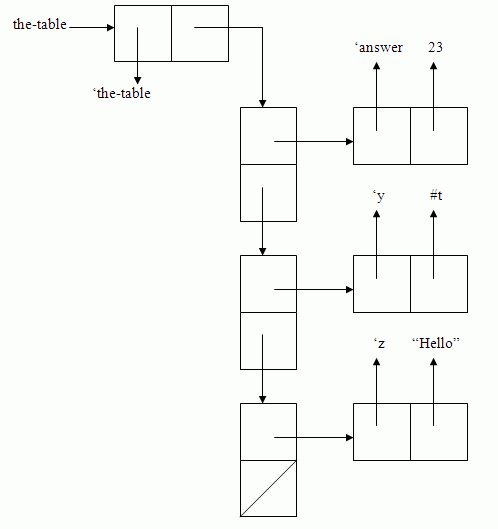To add a new element, set the cdr of the new list element to the pointer from the header pair and then change the header pair pointer to point at the new element.